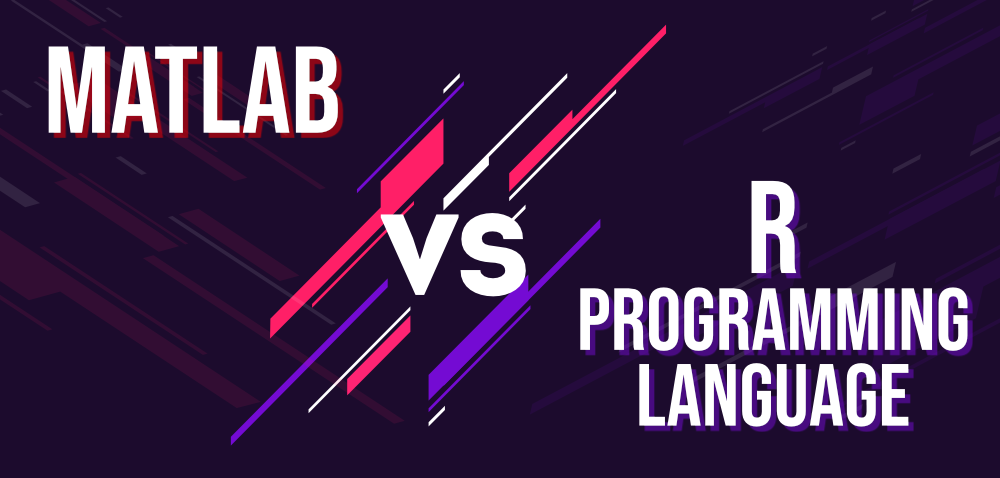Related Articles
Differences Between MATLAB and R Programming Language
• Last Updated : 06 Jul, 2020

MATLAB: MATLAB stands for Matrix Laboratory. MATLAB is a high performance language, initially intended to provide access to the matrix software designed by the projects LINPACK (Linear System Package) and EISPACK (Eigen System Package). It incorporates the environment for computation, visualization and programming. MATLAB provides a modern language programming environment: it has complex data structures, provides built-in editing and debugging software, and bolsters object-oriented programming. It was developed in 1984 by MathWorks. Inc. Company by Cleve Molar. It is written in C, C++, and Java.

• MATLAB, among other programming languages, provides greater visualization of plots or data.
• We can integrate a package with other packages with the help of a single line or a few lines .
• When it comes to numeric calculation or analysis, MATLAB gives quicker results than the other programming languages.

R Programming Language: R is an open source programming language which provides software environment for statistical analysis, graphical depiction and reporting. R is an interpreted computer language which permits branching and looping along with modular programming using functions. For greater precision, it allows integration with the procedures written in the languages C, C++, .Net, Python or FORTRAN. R can be found across commonly deployed systems such as Windows, Linux and MacOS.

• R is the most comprehensive package on statistical analysis. As new technologies and concepts often first appear in R.
• R is a cross-platform programming which can run on any operating system.
• R has a rich set of packages.Below is a table of differences between MATLAB and R Programming Language:

Based onMATLABR Programming Language
Language Type

Matlab is a high-performance language.

R is an interpreted language.

Usage

Matlab is used for other mathematical factors such as calculus, designing graphs, matrix manipulation, signal processing etc.

R is being used to solve statistical-related problems and has many pre-packaged applications that help resolve analytical problems, so R is recommended over Matlab in the field of analytics.

Object-oriented Programming

In Matlab, a class can be specified as a folder or function directory used to work with objects of that class.

R classes are accessible in packages format.

Open-source and Cost

Matlab is an not an open-source. Since Matlab has the license cost attached, the amount of code available online is exiguous.

R is an open-source and has a huge amount of open source codes available online for free.

Operating System

Unless different operating systems are installed on the same physical machine, Matlab cannot run the same licensed version on the different operating systems.

R can run on any operating system such as Windows, Linux and MacOS.

Ease of Use

Matlab is easier to learn, because it has a lot of tool kits for most of the functionalities.

R follows the syntax of programming language thus, making it hard for the newcomer to comprehend.

Performance

When it comes to basic programming functions Matlab is quicker than R. So, it is used in statistics and machine learning.

R is slower than Matlab. But proficient programmer in R can accomplish results faster and boost efficiency.

Support and Documentation

Matlab is licensed. So, it has closed community and documentation for MATLAB is incomparable and exceptional.

As R is an open source language, it has a large developer community for support and documentation.

Machine Learning

Matlab provides statistics as well as machine learning toolbox.

R has extensive library sets for machine learning.

Visualization

To have the graphical user interface, Matlab provides 2D and 3D plotting features.

R offers four different graphic implementations such as Base graphics, Grid graphics, Lattice graphics, and Ggplot2.

My Personal Notes arrow_drop_up